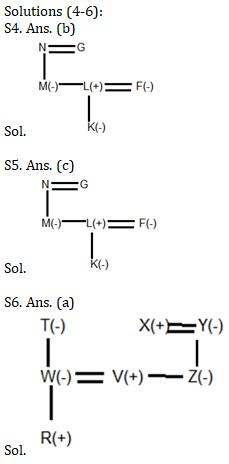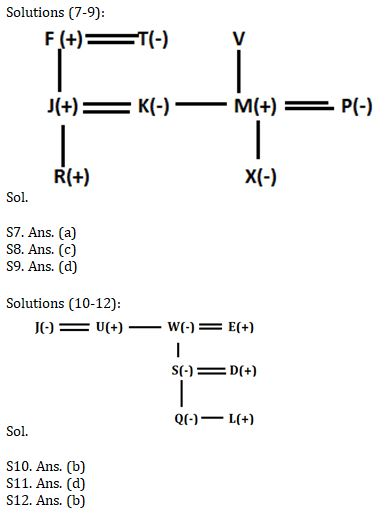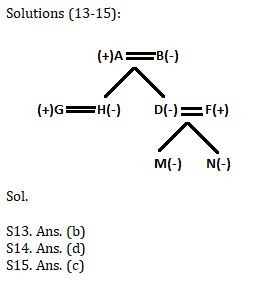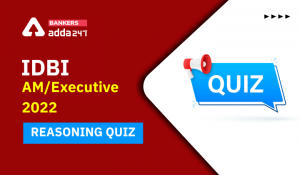Latest Banking jobs   »   Reasoning Ability Quiz For SBI Clerk...

# Reasoning Ability Quiz For SBI Clerk Prelims 2021- 24th May

Directions (1-3): Study the following information carefully and answer the given questions.
A @ B means A is father of B
A % B means A is husband of B
A \$ B means A is sister of B
A ₤ B means A is mother of B
A ¥ B means A is brother of B

Q1. What should come in place of question mark to make the expression “E is grandfather of T” true?
E @ V ? N % R ₤ T
(a) @
(b) ₤
(c) ¥
(d) %
(e) None of these

Q2. Which of the following statements is true if the given expression is definitely true?
A % L \$ K ₤ B ¥ C
(a) K is sister-in-law of A
(b) C is daughter of K
(c) A is father of B
(d) C is son of L
(e) None of these

Q3. Which of the following expressions, if true, makes “N is sister of R” true?
(a) F ₤ U @ N \$ K ¥ R
(b) N \$ F ₤ K ¥ R \$ U
(c) R \$ U ¥ K \$ N
(d) N ₤ F \$ K ¥ R \$ U
(e) None of these

Directions (4-6): Study the following information carefully to answer the given questions
M is daughter of N and sister of L. K is daughter of L and granddaughter of G. F is sister-in-law of M. L is only sibling of M.

Q4.How is K related to N?
(a)Grandson
(b)Granddaughter
(c)Daughter
(d)Daughter-in-law
(e)None of these

Q5. How is F related to G?
(a)Daughter
(b)Mother
(c)Daughter-in-law
(d)Mother-in-law
(e)None of these

Q6. X is married to Y. Y is mother of Z. Z is sister of V. Y has only one daughter. V is married to W. R is son of W. T is mother of W.
How is X related to R ?
(a)Grandfather
(b)Father
(c)Uncle
(d)Father-in-law
(e)None of these

Directions (7-9): Study the following information carefully and answer the questions given below:
K is the daughter-in-law of F. K is the daughter of V. V has two children. R is the grandson of T. F has only one son. T is not married to V. P is the mother of X and married to M. F is the father of J. M is the sibling of K. M has no son.

Q7. If W is the father of M, then how is V related to R?
(a) Grand Mother
(b) Grand Father
(c) Sister
(d) Brother
(e) Husband

Q8. How is P related to K?
(a) Mother
(b) Father-in-law
(c) Sister-in-law
(d) Brother
(e) Husband

Q9. How is X related to K?
(a) Mother
(b) Nephew
(c) Sister
(d) Niece
(e) Husband

Direction (10-12): Study the following information carefully to answer the given questions:
In a family D is the son-in-law of W. U is the brother-in-law of E. S is the only child of E. J is married to U. Q is the granddaughter of W. L is the son of S. W is the wife of E. E has no sister.

Q10. How is S related to U?
(a) Mother
(b) Niece
(c) Uncle
(d) Brother
(e) None of these

Q11. How is L related to Q?
(a) Mother
(b) Sister
(c) Uncle
(d) Brother
(e) None of these

Q12. How is J related to W?
(a) Mother-in-law
(b) Sister-in-law
(c) Uncle
(d) Brother
(e) None of these

Directions (13-15): Study the following information carefully and answer the given questions.
In a family of eight members in which five female members. G is son-in-law of A, who is married to B. B is mother of two daughters. F is brother-in-law of H. D is the mother of M and N. F is a married member of a family.

Q13. How is M related to B?
(a) Grandson
(b) Granddaughter
(c) Son
(d) Either (a) or (b)
(e) Cannot be determined

Q14. How is H related to M?
(a) Grandmother
(b) Mother
(c) Sister
(d) Maternal Aunt
(e) None of these

Q15. How is F related to A?
(a) Son
(b) Brother
(c) Son-in-law
(d) Brother-in-law
(e) None of these

Practice More Questions of Reasoning for Competitive Exams:

###### SBI Clerk Study Plan 2021

Solutions

Solutions (1-3):
Sol. @ → father
% → Husband
\$ → Sister
₤ → mother
¥ → brother

S1. Ans.(c)
S2. Ans.(a)
S3. Ans.(a)#### Congratulations!Incorrect details? Fill the form again here

•Reasoning Ability Quiz For IBPS RRB PO C...
•Reasoning Ability Quiz For IBPS Clerk Pr...
•Reasoning Ability Quiz For IBPS RRB PO P...
•Reasoning Ability Quiz For IDBI AM/Execu...
•Reasoning Ability Quiz For IBPS RRB PO P...
•Reasoning Ability Quiz For IDBI AM/Execu...